Generalizing and Hybridizing Count-based and Neural Language Model

This paper demonstrates a framework called Mixture Of Distribution Language Models (MODLMs), which provides a single mathematical framework that encompasses several widely used classes of LMs.

Introduction

This paper demonstrates a framework called Mixture Of Distribution Language Models (MODLMs), which provides a single mathematical framework that encompasses several widely used classes of LMs. This paper describes two novel ways to combine the desirable features of traditional n-gram model and neural LMs: neural interpolated n-gram LMs, and neural/n-gram hybrid LMs. The authors executed experiments on two corpus: the Penn Treebank (PTB) dataset, and the first 100k sentences on the English side of the ASPEC corpus. [1, 2] After these two trials, they also experimented on the larger data sets (WSJ and GW) and make a comparison with Static Interpolation. The results shows that their new framework has good performance at combining models, and achieved better or similar results compared with existing models. This framework may give us some inspiration on improving the language models.

1. Background：

In case some readers do not know what an N-gram model is, I would like to provide some material which may aid in understanding this paper.

A language model is a function or an algorithm for learning such a function, that captures the salient statistical characteristics of the distribution from a sequence of words in a natural language. Typically, we could query it to get the probability of our “query”. For example, if I have a query “I like”, then I could get the probability of P(X | I like). X could be any word.

1.1 N-gram Language Model & Log Probabilities

First, I would like to give a brief introduction to some basic concepts:

N-gram Language Model

Models that assign probabilities to sequences of words are called language models or LMs. N-gram is the simplest language model. It is a sequence of N words: a 2-gram (bi-gram) is a two-words sequence of words like “please turn”, and a 3-gram (or trigram) is a three-word sequence of words like “please turn your”. “N” means how many words will be treated as a “unit” to get the probability. The probability of word will be related with its preceding N-1 words.

Two things we need to know (at least for this paper):

1. How to use N-gram models to estimate the probability of the last word of an N-gram given the previous words.
2. How to assign probabilities to entire sequences.

(Note: In a bit of terminological ambiguity, we usually drop the word “model”, and thus the term N-gram is used to mean either the word sequence itself, or the predictive model that assigns it a probability.)

Log Probabilities

Why use log probabilities for language model? Since probabilities are (by definition) less than or equal to 1, the more probabilities we multiply together, the smaller the product will become. Multiplying enough N-grams together would result in numerical underflow. By using log probabilities instead of raw probabilities, we get numbers that are not as small. Adding in log space is equivalent to multiplying in linear space, so we combine log probabilities by adding them. The result of doing all computation and storage in log space is that we only need to convert back into probabilities if we need to report them at the end; then we can just take the exponent of the logprob: p1 × p2 × p3 × p4 = exp(log p1 +log p2 +log p3 +log p4)

1.2 Neural Language Model

A neural network language model means every operation you will do with this language model is based on a Neural Network. For example, the probability of your query will be the output of neural network instead of statistic method. There are some discussions in section 3.1. (For more information please refer to [13,14,15])

2. Motivation of this paper:

For count-based LMs, smoothed (because of data sparsity ) n-grams is the most traditional and broadly used language model. Recently, neural networks have outperformed count-based LMS. However, neural LMs are limited with the cost of computational complexity at both training and test time, and even the largest reported neural LMs are trained on a fraction of the data of their count-based counterparts. [4, 5, 6]

This paper focus on mixture of distributions LMs (MODLMs), and they define the following form: calculating the probabilities of the next word in a sentence wi, given preceding context c according to a mixture of several component probability distributions P(wi|c):Where Lambda_k(c) is the function which control the weight for each P_k. And the sum of all the Lambda is 1. Then Equation (1) can be reformed as following matrix-vector multiplication:Where Pc is a vector with length equal to vocabulary size. This formula could be understood according to the Figure 1.Depending on the formulation above, this paper creates novel methods to combine the desirable features of count-based and neural LMs.

3. MODLMs：

3.1 Existing LMs as Linear Mixtures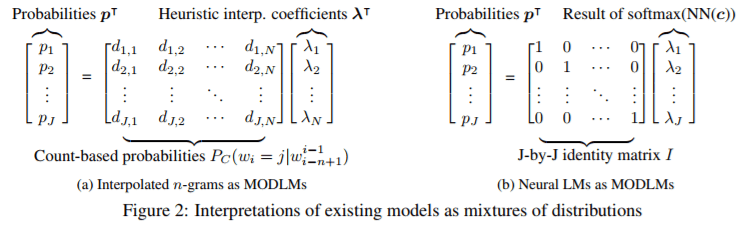N-gram LMs as Mixtures of Distributions

This paper offers the explanations of Maximum likelihood estimation (ML), interpolation and discounting. [7, 8]

As we all know, the common method to calculate probability is Maximum likelihood estimation (ML):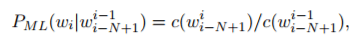Where c counts frequency in the training corpus

Because of data sparsity, ML may assign zero (close to zero) to word sequences whose counts are very small or equal to zero. Interpolation is a common way to address this problem.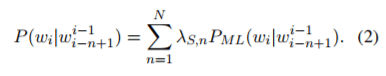(as shown in Figure 2 a.)

Using heuristics based on the frequency counts of the context to calculate λ(c) dynamically could improve the performance:(Where is a context-sensitive fall-back probability for order n models)

The other widely used technique is discounting, which defines a fixed discount d and subtracts it from the count of each word before calculating probabilities:A pretty good explanation about smooth techniques (Additive smoothing, Good-Turing estimate, Jelinek-Mercer smoothing (interpolation), Katz smoothing (backoff), Witten-Bell smoothing, Absolute discounting, Kneser-Ney smoothing) can be found at 

Neural LMs as Mixtures of Distributions

This paper offers the explanation of Feed-forward neural network LMs and Recurrent neural network LMs.

P=softmax(hW+b) (where h is the output of previous affine layers)

For neural LMs (both feed-forward and RNN), instead of calculating p directly, it calculates mixture weights λ=(hW+b) and defines the MODLM’s distribution matrix D as a J-by-J identity matrix. (Figure 2b)

3.2 Novel Application of MODLMSNeurally Interpolated n-gram Models

Based on exiting methods described in last subsection, instead of using heuristic interp. cefficients λ, we use the method described in 3.1 to replace the Heuristic interp. coefficients in Figure 2a, then we got Figure 3a.

Neural/n-gram Hybrid Models

It is similar to the last one. But matrix is augmented with J-by-J identity matrix, which is showed in Figure 3b. I do think this figure is clear for readers.

This method access all information used by both neural and n-gram LMs, and really combine the results of n-gram and neural network like a blender. Intuitively, the more information this model can capture, the better performance this model can reach. And the results of later experiments showed this.

(This paper describe interpolation on NMT similar to this paper:

4. Learning and Block Dropout:

Training objective:

The training objective here is a negative log-likelihood loss summed over words wi in every sentence w in corpus W:Where c represents all words preceding wi in w that are used in the probability calculation. Apart from this training objectives, others are like standard neural network LMs.

Block dropout for Hybrid Models

In Neural/n-gram Hybrid Models, the probabilities of n-gram are already good, and the weights of J-by-J matrix are not yet able to provide accurate probabilities, so this model will learn to set the mixture proportions to near zero and rely mainly on the count-based n-gram distribution.

Ammar et al. (2016) proposed a method called block dropout which randomly drop out subsets of entire network nodes (standard dropout  drops single nodes, they are different processing units). Therefore, for a random block of the whole training data (they use 50% here), they disable the part of n-gram by zeroing out all elements in λ(c) that correspond to n-gram distributions to make the whole model rely on J-by-J matrix only.

5. Results:

The results of the experiments showed the following:

First, they choose two relatively small data sets: PTB and ASPEC. Table 1 shows the details of these two datasets. Table 2 shows the results of Neurally Interpolated n-grams.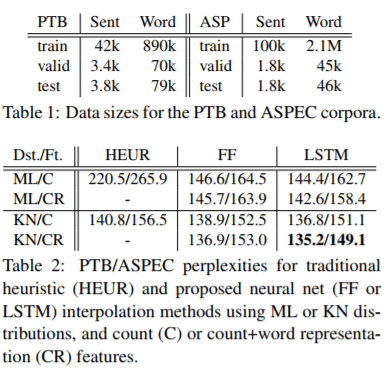As described in the caption of Table 2, there are actually 10 different combinations among these techniques. (ML: Maximum likelihood estimation, KN: Kneser-Ney, C: count, CR: count + word, HEUR: heuristic, FF: feed-forward neural network, RNN (LSTM) E.g. 220.5/265.9 is PTB/ASPEC. ML/C or ML/CR is ML with different features.Table 3 is the result of Neural/n-gram Hybrids. The δ in the table is Kronecker δ distributions, which are equivalent to J-by-J identity matrix we mentioned above.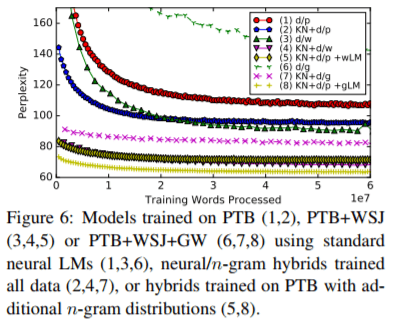Figure 6 shows their results on larger datasets WSJ and GW. It is a little dazzling. All in all, the results showed neural/n-gram hybrids outperform the traditional neural LMs. The type and size of the data also affects the performance of method. For the WSJ data, training on all data slightly outperforms the method of adding distributions, but when the GW data is added, this trend reverses. Therefore, the choice of domain-specific interpolation is also important for different data set.

Finally, they combined the independently trained models with static interpolation weights tuned on the validation set using the EM algorithm, which is what Lin. represents in the form. This was done with the “interp-probs” program in the modlm toolkit [https://github.com/neubig/modlm]. “+WSJ” means that they additionally added the large WSJ data. (Thanks to Graham Neubig’s help)Table 4 shows the results of comparison with Static Interpolation. The results show that the proposed method could get a similar or better performance.

Conclusion and personal opinion:

This paper proposed a framework for a language model which could combine the desirable features of different language models. Since language model can be used in many related fields, such as machine translation and automatic speech recognition ,and this is a new way to combine different technologies (neural network and statistic method here), it may invoke a new trend to improve existing technology.

Reference (apart from papers I mentioned above):

 Tomas Mikolov, Martin Karafiat, Lukas Burget, Jan Cernocky, and Sanjeev Khudanpur. 2010. Recurrent neural network based language model. In Proc. InterSpeech,pages 1045–1048.

 Toshiaki Nakazawa, Hideya Mino, Isao Goto, Graham Neubig, Sadao Kurohashi, and Eiichiro Sumita. 2015. Overview of the 2nd Workshop on Asian Translation. In Proc. WAT

 1,S. F. Chen and J. Goodman. 1999. An empirical study of smoothing techniques for language modeling. Computer Speech & Language, 13(4):359–393.

 Chen, Welin, David Grangier, and Michael Auli. “Strategies for training large vocabulary neural language models.” arXiv preprint arXiv:1512.04906 (2015).

 Williams, Will, et al. “Scaling recurrent neural network language models.” Acoustics, Speech and Signal Processing (ICASSP), 2015 IEEE International Conference on. IEEE, 2015.

 Brants, Thorsten, et al. “Large language models in machine translation.” U.S. Patent No. 8,332,207. 11 Dec. 2012.

 Ney, Hermann, Ute Essen, and Reinhard Kneser. “On structuring probabilistic dependences in stochastic language modelling.” Computer Speech & Language 8.1 (1994): 1-38.

 Arthur, Philip, Graham Neubig, and Satoshi Nakamura. “Incorporating discrete translation lexicons into neural machine translation.” arXiv preprint arXiv:1606.02006 (2016).

 Ammar, Waleed, et al. “Many languages, one parser.” arXiv preprint arXiv:1602.01595 (2016).

 Srivastava, Nitish, et al. “Dropout: a simple way to prevent neural networks from overfitting.” Journal of Machine Learning Research 15.1 (2014): 1929-1958.

Author: Junyi | Reviewer: Haojin Yang